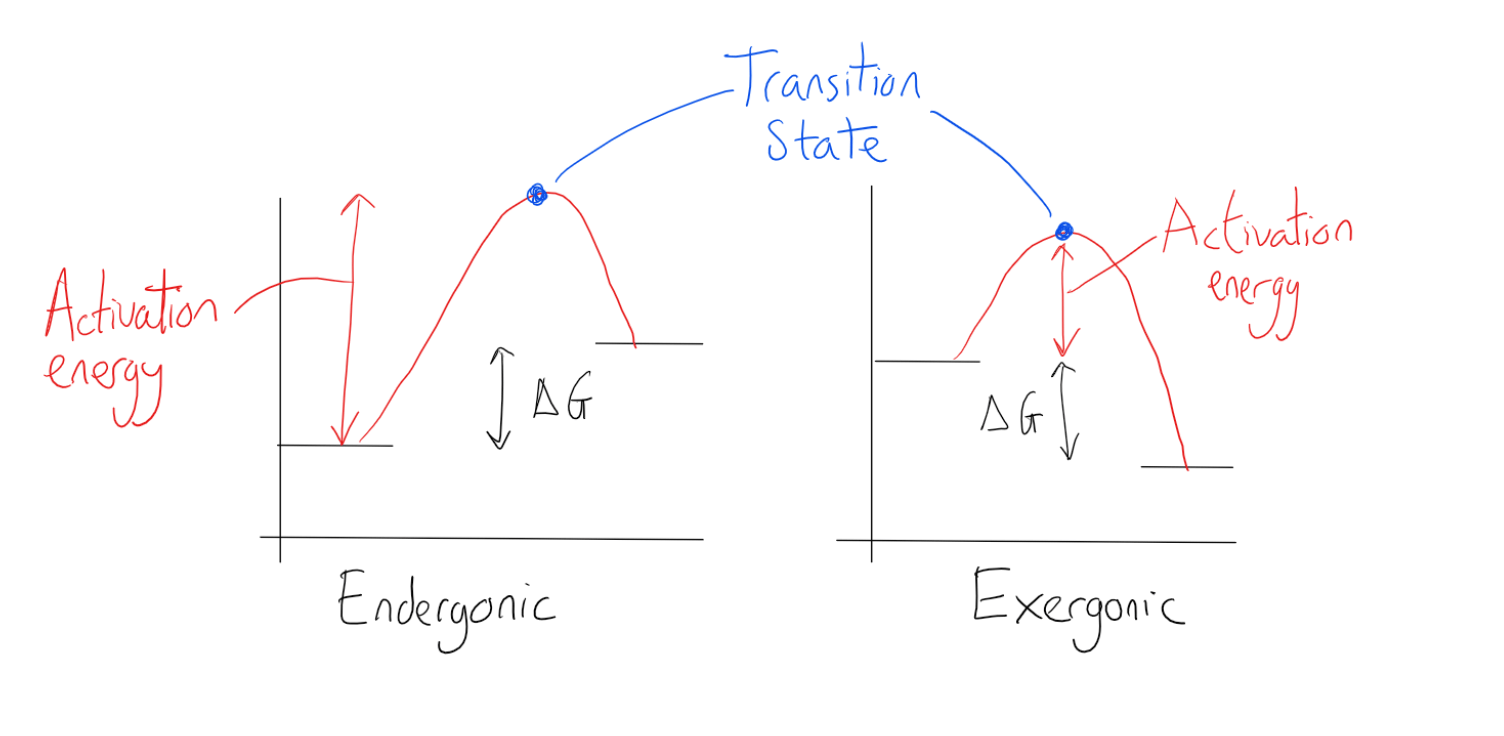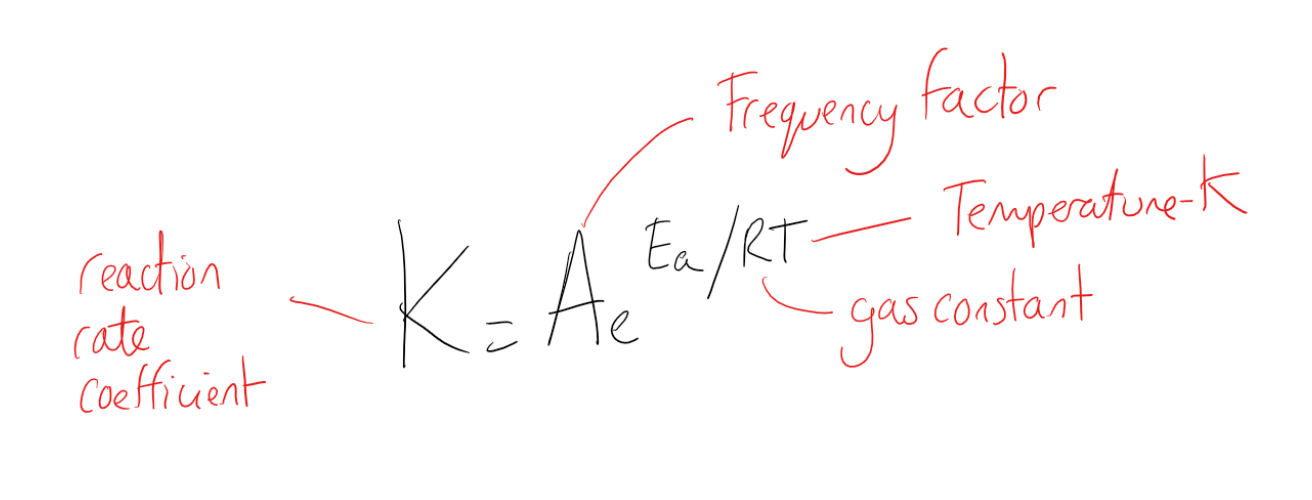Complimentary 1-hour tutoring consultation

MCAT Content / Rate Processes In Chemical Reactions Kinetics And Equilibrium / Dependence Of Reaction Rate Upon Temperature

### Dependence of Reaction Rate Upon Temperature

Topic: Rate Processes In Chemical Reactions Kinetics And Equilibrium

Many chemical reactions and almost all biochemical reactions do not occur spontaneously and must have an initial input of energy (called the activation energy) to get started.

Reaction with a negative ∆G reaction requires some energy to proceed. Activation energy must be put in to achieve the complex or transition state: it is a high-energy, unstable state where chemical bonds have been contorted and sometimes broken. For this reason, reactant molecules don’t last long in their transition state, but very quickly proceed to the next steps of the chemical reaction. The activation energy of a particular reaction determines the rate at which it will proceed. The higher the activation energy, the slower the chemical reaction will be.

When considering the rate of reaction, activation energy, and the temperature, it can be seen that as temperature increases, molecules gain energy and move faster and faster. Therefore, the greater the temperature, the higher the probability that molecules will be moving with the necessary activation energy for a reaction to occur upon collision.

Free energy diagrams illustrate the energy profiles for a given reaction. Whether the reaction is exergonic (ΔG<0) or endergonic (ΔG>0) determines whether the products in the diagram will exist at a lower or higher energy state than the reactants. However, the measure of the activation energy is independent of the reaction’s ΔG. The activation energy can be represented as the amount of energy required to get from the reactants to the transition state.The source of the activation energy needed to push reactions forward is typically heat energy from the surroundings. Heat energy (the total bond energy of reactants or products in a chemical reaction) speeds up the motion of molecules, increasing the frequency and force with which they collide. It also moves atoms and bonds within the molecule slightly, helping them reach their transition state. For this reason, heating a system will cause chemical reactants within that system to react more frequently. Increasing the pressure on a system has the same effect. Once reactants have absorbed enough heat energy from their surroundings to reach the transition state, the reaction will proceed.

The Arrhenius equation relates the rate of a chemical reaction to the magnitude of the activation energy:The Arrhenius equation can be written in a non-exponential form, which is often more convenient to use and to interpret graphically. Taking the natural logarithms of both sides and separating the exponential and pre-exponential terms yields:

ln(k)=ln(A)−EaRT

Note that this equation is of the form:

y=mx+b

Thus creating a plot of ln(k) versus 1/T will produce a straight line with the slope –Ea /R from which activation energy of a reaction can be calculated.

Practice Questions

Basic concepts in bioenergetics: phosphoryl group transfers and ATP hydrolysis

Purification of caffeine

MCAT Official Prep (AAMC)

Chemistry Question Pack Passage 10 Question 58

Chemistry Question Pack Passage 11 Question 61

Practice Exam 1 B/B Section Passage 3 Question 16

Practice Exam 3 B/B Section Passage 8 Question 44

Practice Exam 3 B/B Section Question 47

Key Points

• Reactions require an input of energy to initiate the reaction; this is called the activation energy (EA).

• Activation energy is the amount of energy required to reach the transition state.

• The source of the activation energy needed to push reactions forward is typically heat energy from the surroundings.

• The Arrhenius equations relate the rate of a chemical reaction to the magnitude of the activation energy and can be calculated from plotting ln K against 1/T

• As the temperature rises, molecules move faster and collide more vigorously, greatly increasing the likelihood of bond breakage upon collision.

Key Terms

Activation energy: The minimum energy required for a reaction to occur.

Catalysis: The increase in the rate of a chemical reaction by lowering its activation energy.

Transition state: An intermediate state during a chemical reaction that has higher energy than the reactants or the products.

Thermodynamic free energy: The change in the free energy is the maximum amount of work that a thermodynamic system can perform in a process at constant temperature, and its sign indicates whether a process is thermodynamically favorable or forbidden.

Exergonic: a chemical reaction where the change in the free energy is negative (there is a net release of free energy), indicating a spontaneous reaction.

Endergonic: Also called a heat absorb nonspontaneous reaction or an unfavorable reaction) is a chemical reaction in which the standard change in free energy is positive, and an additional driving force is needed to perform this reaction. In layman’s terms, the total amount of useful energy is negative (it takes more energy to start the reaction than what you get out of it) so the total energy is a negative net result.

Arrhenius equation: A formula for the temperature dependence of reaction rates.

Billing Information